# Circle Sectors, Segments and Arcs

GCSELevel 4-5AQAEdexcelOCR

## Circle Sectors, Segments and Arcs

It can be useful to calculate the area and arc length of sectors and segments of circles.

Make sure you are happy with the following topics before continuing:

Level 4-5GCSEAQAEdexcelOCR

## Area of a Sector

$\textcolor{blue}{\text{Area of a sector}} = \dfrac{\textcolor{limegreen}{x}}{360} \times \textcolor{red}{\text{Area of full circle}}$

Where $\textcolor{limegreen}{x}$ is the angle of the sector.

$\textcolor{red}{\text{area of the circle}} = \textcolor{red}{\pi r^2}$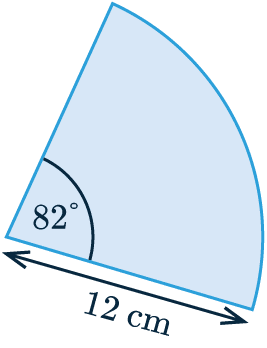Calculating the area of the circle sector shown involves using the angle of the sector $\textcolor{limegreen}{82}$ and the radius $\textcolor{red}{12}$ cm.

We apply these to the equation above:

$\dfrac{\textcolor{limegreen}{82}}{360} \times \textcolor{red}{\pi \times 12^2} = 103.04 \text{ cm}^2$

Level 4-5GCSEAQAEdexcelOCR

## Area of a Segment

$\textcolor{blue}{\text{Area of a segment}} = \dfrac{\textcolor{limegreen}{x}}{360} \times \textcolor{red}{\text{Area of full circle}} - \textcolor{purple}{\text{Area of triangle}}$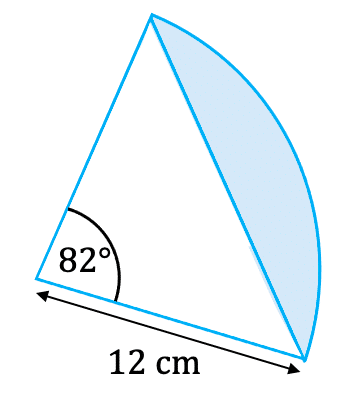Where $\textcolor{limegreen}{x}$ is the angle of the sector.

$\textcolor{red}{\text{area of the circle}} = \textcolor{red}{\pi r^2}$

$\textcolor{purple}{\text{area of the triangle}} = \textcolor{purple}{\dfrac{1}{2}\,ab\, \text{sin} C}$

Calculating the area of the circle segment shown involves using the angle of the sector $\textcolor{limegreen}{82\degree}$ and the radius $\textcolor{red}{12}$ cm.

We apply these to the equation above:

$\dfrac{\textcolor{limegreen}{82}}{360} \times \textcolor{red}{\pi \times 12^2} - \textcolor{purple}{\dfrac{1}{2} \times 12 \times 12 \times \text{sin} 82}= 31.74 \text{ cm}^2$

Click here to practice how to find the area of non-right angle triangles.

Level 4-5GCSEAQAEdexcelOCR

## Arc Length

The arc length is the length of the part of the circumference which is part of the circle sector. The equation for this is:

$\textcolor{purple}{\text{Arc Length}} = \dfrac{\textcolor{limegreen}{x}}{360\degree} \times \textcolor{red}{\text{circumference of full circle}}$

Where $\textcolor{limegreen}{x}$ is the angle of the sector.

$\textcolor{red}{\text{Circumference of the full circle}} = \textcolor{red}{\pi D}$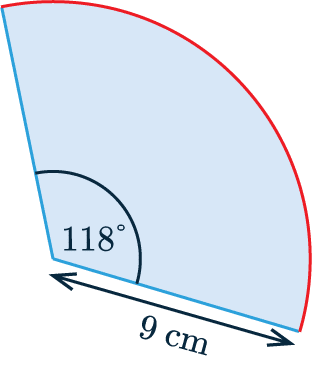We can calculate the arc length of the circle sector shown by using the angle of the sector $\textcolor{limegreen}{118\degree}$ and the diameter $2 \times\textcolor{red}{9} = \textcolor{red}{18}$ cm.

Now we apply these to the equation above:

$\dfrac{\textcolor{limegreen}{118\degree}}{360\degree} \times \textcolor{red}{\pi \times 18} = 18.54$ cm

Level 4-5GCSEAQAEdexcelOCR
Level 4-5GCSEAQAEdexcelOCR

## Example 1: Area and Circumference

The circle shown has centre $C$.

Find its area and circumference to $1$ decimal place.

[2 marks]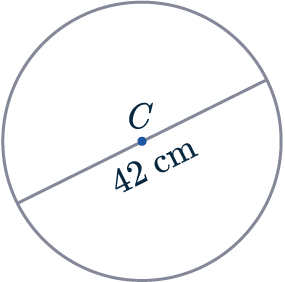As the line passes through the centre, we know it is a diameter of the circle. So:

Circumference $= \pi d = \pi \times 42 = 131.9 \text{cm (1 dp)}$

The diameter is twice the length of the radius, so the $\text{radius } = 42 \div 2 = 21$cm. So:

Area of circle $= \pi r^2=\pi\times (21^2) = 1385.4\text{cm}^2\text{ (1 dp)}$

Note: questions may ask you to “leave your answer in terms of $\pi$”, this means that the answer should be in the form of “something $\times \pi$”.

Level 4-5GCSEAQAEdexcelOCR

## Example 2: Sector Area & Arc Length

The sector of a circle has centre $C$ as shown.

Find the area of the sector and the arc length to $1$ decimal place.

[2 marks]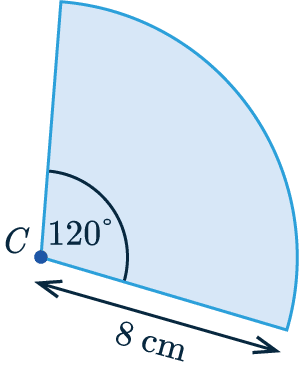The angle is $120 \degree$, which means that this sector is $\frac{120}{360}$ as a fraction of the whole circle.

So, we get:

$\textcolor{blue}{\text{Sector Area}} = \dfrac{120}{360} \times \pi \times 8^2$

$= 67.0\text{ cm}^2$ ($1$ dp)

$\textcolor{purple}{\text{Arc Length}} = \dfrac{120}{360} \times \pi \times 2 \times 8$

$= 16.8\text{ cm}$ ($1$ dp)

Level 4-5GCSEAQAEdexcelOCR

## Example 3: Segment Area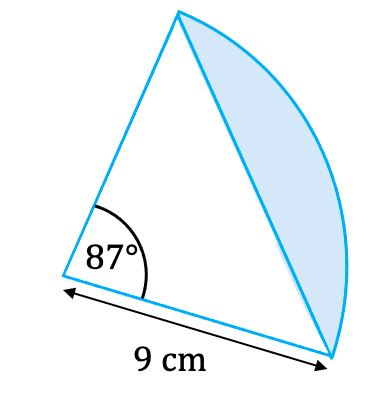The sector of a circle has centre $C$ as shown.

Find the area of the segment to $1$ decimal place.

[2 marks]

For the question, we have a sector with an angle of $87 \degree$ and a radius of $9 \text{ cm}$. To find the area of the segment highlighted we do the following:

$\dfrac{\textcolor{limegreen}{87}}{360} \times \textcolor{red}{\pi \times 9^2} - \textcolor{purple}{\dfrac{1}{2} \times 9 \times 9 \times \text{sin} 87}= 21.1 \text{ cm}^2$

Level 4-5GCSEAQAEdexcelOCR

## Circle Sectors, Segments and Arcs Example Questions

The formula for the area of a circle is $\pi r^2$. In this question we are given the diameter rather than the radius.  Given that the diameter is twice the length of the radius:

$\text{radius} = 5.24 \div 2 = 2.62 \text{cm}$

Therefore,

$\text{area } = \pi r^2 = \pi \times (2.62)^2 = 21.6 \text{cm}^2$Gold Standard Education

The formula for the area of a circle is $\pi r^2$. The area of the sector is,

$\text{area} =\dfrac{72\degree}{360\degree} \times \pi (5)^2 = \dfrac{72\degree}{360\degree} \times 25 \pi = 5\pi\text{ m}^2$Gold Standard Education

The formula for the area of a circle is $\pi r^2$. The area of this sector, $26.15 \text{m}^2$, must be equal to $\frac{x}{360}$ of the total area of the circle. So, as an equation, this looks like:

$26.15 = \dfrac{x\degree}{360\degree} \times \pi \times 9^2$

$26.15=\dfrac{x\degree}{360\degree} \times 81\pi$

Dividing the left-hand side and right-hand side by $81 \pi$ gives

$\dfrac{26.15}{81\pi} = \dfrac{x\degree}{360\degree}$

Then, multiply both sides by 360 to get,

$x=\dfrac{26.15}{81\pi}\times 360\degree$

$x = 37.0\degree$ (to 1d.p.)Gold Standard Education

The question asks for the total perimeter of the shape. We know that one side is 14 mm but the other two are missing. Immediately we can identify that the other straight side is also 14 mm. Then, all that remains is to calculate the arc length.

The angle in this sector is 165 degrees, meaning that the arc length will be equal to $\frac{165}{360}$ of the total circumference. The formula for the circumference is $\pi d$, or alternatively (and more helpfully in this case), $2\pi r$. So, we get:

Arc length $= \dfrac{165}{360} \times 2 \pi \times 14 = 40.3 \text{mm}$

Therefore,

$\text{total perimeter} = 14 + 14 + 40.3 = 68.3 \text{mm}$Gold Standard Education

The formula for the area of a circle is $\pi r^2$. The area of this sector, $160 \text{m}^2$, must be equal to $\frac{x}{360}$ of the total area of the circle. So, as an equation, this looks like:

$160 = \dfrac{x\degree}{360\degree} \times \pi \times 9^2$

$160=\dfrac{x\degree}{360\degree} \times 81\pi$

Dividing the left-hand side and right-hand side by $81 \pi$ gives

$\dfrac{160}{81\pi} = \dfrac{x\degree}{360\degree}$

Then, multiply both sides by 360 to get,

$x=\dfrac{160}{81\pi}\times 360\degree$

$x = 226\degree$ (to 3s.f.)Gold Standard Education

Since the distance between $C$ and $D$ is $10 \text{ cm}$, we know the radius of each circle is also $10\text{ cm}$.

The region where the two circles overlap is created by two segments of equal sizes.

Area of region $=2\times(\text{Area of sector} - \text{Area of triangle})$

Area of region $=2\times \left(\dfrac{120}{360}\times \pi \times 10^2 - \dfrac{1}{2}\times 10 \times 10 \times \text{ sin} 120 \right) = 122.84 \text{ cm}^2$Gold Standard Education

## Circle Sectors, Segments and Arcs Worksheet and Example Questions

### (NEW) Circle Sector, Segments and Arcs Exam Style Questions - MME

Level 4-5GCSENewOfficial MME

## Circle Sectors, Segments and Arcs Drill Questions

### Area of Circles & Segments

Level 4-5GCSEGold Standard Education

£19.99 /month. Cancel anytime

Level 4-5GCSEKS3